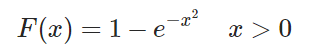# Suppose the cumulative distribution function of the random variable x is

Suppose that the cumulative distribution function of the random variable X is given byEvaluate (a) P{X>2}; (b) P{1<X<3}; © the hazard rate function of F; (d) E[X]; (e) Var(X).